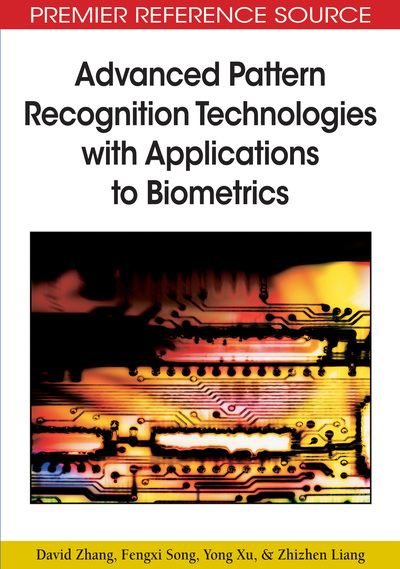# Tensor Principal Component Analysis

David Zhang (Hong Kong Polytechnic University, Hong Kong), Fengxi Song (New Star Research Institute Of Applied Technology, China), Yong Xu (Harbin Institute of Technology, China) and Zhizhen Liang (Shanghai Jiao Tong University, China)
DOI: 10.4018/978-1-60566-200-8.ch008
Available
\$37.50
No Current Special Offers

## Abstract

Tensor principal component analysis (PCA) is an effective method for data reconstruction and recognition. In this chapter, some variants of classical PCA are introduced and the properties of tensor PCA are analyzed. Section 8.1 gives the background and development of tensor PCA. Section 8.2 introduces tensor PCA. Section 8.3 discusses some potential applications of tensor PCA in biometrics. Finally, we summarize this chapter in Section 8.4.
Chapter Preview
Top

## Introduction

Principal component analysis (Turk & Pentland, 1991; Penev & Sirovich, 2000), also known as Karhunen-Loève (K-L) transform, is a classical statistical technique that has been widely used in various fields, such as face recognition, character recognition, and knowledge representation. The aim of PCA is to reduce the dimensionality of the data so that the extracted features are representative as possible. In general, the key idea of PCA is to project data to an orthogonal subspace, which can transform correlated variables into a smaller number of uncorrelated variables. The first principal component can capture variance of the data along some direction as possible, and consequent components capture as much of the remaining variability as possible. Up to now, there are a number of theoretical analyses and discussion for PCA in the literature and PCA is one of the most popular methods for data representation.

In recent years, some researchers (Yang, Zhang, Frangi, & Yang, 2004; Ye, 2004) noted that classical PCA often runs up against computational limits due to the high time and space complexity for dealing with large image matrices, especially for images and videos. In applying PCA, data must be converted to a vector form. This results in the difficulty in eigen-decomposition in a high dimensional vector space. To overcome this limitation, a novel idea is developed. This novel idea lies in dealing with image matrices or video data directly rather than converting them into vectors prior to dimensionality reduction. Based on this, Yang, Zhang, Frangi and Yang (2004) proposed a two-dimensional PCA for image representation, whose idea is that 2D image matrices are used to directly construct the image covariance matrix. This improves the computational efficiency. Moreover, the projection of sample on each principal orthogonal vector is a vector. A drawback of 2DPCA is that it needs more coefficients than PCA for image representation and costs more time to calculate distance in classification phase. In order to address this problem, Ye (2004) proposed a new algorithm called generalized low rank approximations of matrices (GLRAM) to reduce the computational cost. Then some researchers proposed a non-iterative algorithm for GLRAM (Liang & Shi, 2005; Liang, Zhang, & Shi, 2007). Moreover, they reveal the optimal property of GLRAM and show that the reconstruction errors of GLRAM are not smaller than those of PCA when considering the same dimension. Likewise, their method is proved to have much less computational time than the traditional singular value decomposition (SVD) technique. In addition, researchers also developed a number of variants of 2DPCA (Xu et al., 2005; Nhat & Lee, 2005; Xu, Jin, Jiang, & Guo, 2006; Hou, Gao, Pan, & Zhang, 2006; Vasilescu & Terzopoulos, 2002; Wang & Ahuja, 2004; Zuo Wang, & Zhang, 2005a, 2005b). In fact, the methods mentioned above belong to the framework proposed by Lathauwer and his partners (Lathauwer, 1997; Lathauwer, Moor, & Vandewalle, 2000a). In 2000, a multilinear generalization of the singular value decomposition was further proposed (Lathauwer, Moor, & Vandewalle, 2000b). Moreover, they also analyzed some properties of the matrix and the higher-order tensor decompositions. Yu and Bennamoun (2006) also proposed nD-PCA algorithm which exploits higher-order singular value decomposition. All the methods contribute to the development of the tensor PCA.

## Complete Chapter List

Search this Book:
Reset